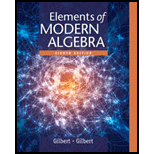# Let f : A → B , where A and B are nonempty. Prove that f ( S 1 ∪ S 2 ) = f ( S 1 ) ∪ f ( S 2 ) for all subsets S 1 and S 2 of A . Prove that f ( S 1 ∩ S 2 ) ⊆ f ( S 1 ) ∩ f ( S 2 ) for all subsets S 1 and S 2 of A . Give an example where there are subsets S 1 and S 2 of A such that f ( S 1 ∩ S 2 ) ≠ f ( S 1 ) ∩ f ( S 2 ) . Prove that f ( S 1 ) − f ( S 2 ) ⊆ f ( S 1 − S 2 ) for all subsets S 1 and S 2 of A . Give an example where there are subsets S 1 and S 2 of A such that f ( S 1 ) − f ( S 2 ) ≠ f ( S 1 − S 2 ) .### Elements Of Modern Algebra

8th Edition
Gilbert + 2 others
Publisher: Cengage Learning,
ISBN: 9781285463230### Elements Of Modern Algebra

8th Edition
Gilbert + 2 others
Publisher: Cengage Learning,
ISBN: 9781285463230

#### Solutions

Chapter
Section
Chapter 1.2, Problem 24E
Textbook Problem

## Expert Solution

### Want to see the full answer?

Check out a sample textbook solution.See solution

### Want to see this answer and more?

Experts are waiting 24/7 to provide step-by-step solutions in as fast as 30 minutes!*

See Solution

*Response times vary by subject and question complexity. Median response time is 34 minutes and may be longer for new subjects.